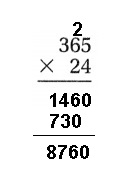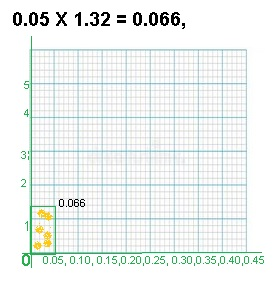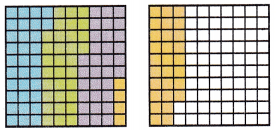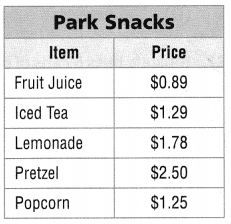Refer to our Texas Go Math Grade 5 Answer Key Pdf to score good marks in the exams. Test yourself by practicing the problems from Texas Go Math Grade 5 Unit 1 Assessment Answer Key.

Vocabulary

Choose the best term from the box.

Vocabulary
Associative Property
Commutative Property
inverse operations

Question 1.
The _________ states that changing the grouping of factors does not change the product. (p. 5)
Commutative Property,

Explanation:
Commutative property is applicable only for addition and multiplication processes.
Thus, it means we can change the position or swap the numbers when adding or
multiplying any two numbers. This is one of the major properties of integers.
For example: 1+2 = 2+1 and 2 x 3 = 3 x 2.
therefore,  the ___Commutative Property__ states that changing the grouping of
factors does not change the product.

Addition and subtraction are ___________. (p. 41)
Inverse operations,

Explanation:
Addition and subtraction are the inverse operations of each other.
Simply, this means that they are the opposite.
We can undo an addition through subtraction and
we can undo a subtraction through addition.

Concepts and Skills

Compare. Write <, >, or =. (TEKS 5.2.B)

Question 3.
6.350.695
6.35 > 0.695,

Explanation:
Given to compare between 6.35 and 0.695
as 6.35 is greater than 0.695 therefore
6.35 > 0.695.

Question 4.
0.020.020
0.02 = 0.020,

Explanation:
Given to compare between 0.02 and 0.020 as
0.02 is equal to 0.020 therefore 0.02 = 0.020.

0.1320.2
0.132 < 0.2,

Explanation:
Given to compare between 0.132 and 0.2
as 0.132 is less than 0.2 therefore 0.132 < 0.2.

Estimate. Then solve. (TEKS 5.3.A. 5.3.B, 5.3.C)

Question 6.
Estimate: _____9,000_______8760, Estimate is 9,000,

Explanation:
Given to multiply 24 with 365 we get 8760 as shown above,
as per estimation 24 ≈ 25 and 365 ≈ 360 we get 25 X  360 = 9,000.

Question 7.
Estimate: ______30______
616 ÷ 22
22)616(28
44_
176
176
0

28, Estimate is 30,

Explanation:
Given to find 616 ÷ 22 we get 28 as
per estimation 616 ≈ 600 and 22 ≈ 20,
now we divide 600 ÷ 20 = 30.

Question 8.
Estimate: ____250_________
5,184 ÷ 18
18)5184(288
36
158
144
144
144
0
288, Estimate is 250

Explanation:
Given to find 5,184 ÷ 18 we get 288 as
per estimation 5,184 ≈ 5,200 and  ≈ 20,
now we divide 5000 ÷ 20 = 250.

Use models or strategies to find the product. Show your work. (TEKS 5.3.D, 5.3.E)

Grade 5 Unit 1 Assessment Answer Key Texas Go Math Question 9.
0.05 × 1.320.05 X 1.32 = 0.066,

Explanation:
Given to find 0.05 X 1.32 if we multiply
11
1.32
X0.05
0.0660 the result 0.0660 shaded region is
shown in the graph.

Question 10.
23 × 5.28
23 × 5.28 = 121.44,

Explanation:
Upon multiplying 23 X 5.28 we get
2
23
X5.28
001.84
004.60
115.00
11
121.44

Question 11.
4.2 × 14.85
4.2 × 14.85 = 62.37,

Explanation:
Given to find 4.2 X 14.85 we get
4.2
14.85
00.21
03.36
16.80
42.00
11
62.37

Use models or strategies to find the quotient. Show your work. (TEKS 5.3.F, 5.3.G)

Question 12.
3.6 ÷ 4
3.6 ÷ 4 = 0.9,

Explanation:
4)3.6(0.9
3.6
0
Upon dividing 3.6 ÷ 4 we get 0.9.

Texas Go Math Grade 5 Answer Key Pdf Unit 1 Question 13.
16.24 ÷ 29
16.24 ÷ 29 = 0.56,

Explanation:
29)16.24(0.56
14.50
1.74
1.74
0

Upon dividing 16.24 ÷ 29 we get 0.56.

Question 14.
96.72 ÷ 62
96.72 ÷ 62 = 1.56,

Explanation:
62)96.72(1.56
62
34.72
31.00
3.72
3.72
0

Question 15.
Kaya’s score in the gymnastics competition is 15.4 when rounded to the nearest tenth.
Which of the following is her actual score? (TEKS 5.2.C)
(A) 15.333
(B) 15.496
(C) 15.395
(D) 15.349
Kaya’s actual score is (C) 15.395,

Explanation:
Given Kaya’s score in the gymnastics competition is 15.4,
when rounded to the nearest tenth her actual score out of
15.333, 15.496, 15.395, 15.349 would be 15.395 as we see
15.4 is near to and in between 15.333<15.349<15.395 and 15.496.

Question 16.
A bakery uses 1,750 kilograms of flour to make 1,000 loaves of bread.
How much flour is needed to make 10 loaves? (TEKS 5.3.G)
(A) 17.5 kilograms
(B) 175,000 kilograms
(C) 1.75 kilograms
(D) 175 kilograms
(A) 17.5 kilograms,

Explanation:
Given a bakery uses 1,750 kilograms of flour to make 1,000 loaves of bread
now flour needed to make 1 loaves is 1,750 kilograms ÷ 1,000 = 1.75 kilograms,
flour needed to make 10 loaves is 1.75 kilograms X 10 = 17.5 kilograms,
therefore matches with (A) 17.5 kilograms.

Question 17.
Maxine paints a mural that is 4.65 meters long.
The width of the mural is 0.8 times the length.
Maxine increases the width by another 0.5 meters.
How wide is the mural? (TEKS 5.3.E, 5.3.K)
(A) 3.77 meters
(B) 37.2 meters
(C) 4.22 meters
(D) 3.72 meters
(D) 3.72 meters,

Explanation:
Given Maxine paints a mural that is 4.65 meters long.
The width of the mural is 0.8 times the length.
So width is 4.65 X 0.8 = 3.72 meters, if Maxine increases
the width by another 0.5 meters then wide the mural is
3.72 X 0.5 meters = 3.72 meters + 1.86 meters = 3.72 meters
which matches with (D).

5th Grade Unit 1 Math Test Texas Go Math Question 18.
Juan uses the model below to solve a problem.
Which of the following equations matches Juan’s model? (TEKS 5.3.F)(A) 0.4 × 32 = 12.8
(B) 1.28 ÷ 4 = 0.32
(C) 0.32 ÷ 4 = 0.08
(D) 0.32 + 4 = 4.32
(A) 0.4 × 32 = 12.8,

Explanation:
Given Juan uses the 2 graph models of 10 X 10 above to solve
the problem the first graph if we count has 32 boxes of 0.4 each,
and the result  is 12.8 boxes therefore the equation is
(A) 0.4 × 32 = 12.8.

Question 19.
The price of a shirt is $26.50. The matching shorts are 0.9 times the price of the shirt. If Li wants to buy the shirt and the shorts, how much money will he need? (TEKS 5.3.E, 5.3.K) (A)$238.50
(B) $35.50 (C)$23.85
(D) $50.35 Answer: (D)$50.35,

Explanation:
Given the price of a shirt is $26.50. The matching shorts are 0.9 times the price of the shirt. So price of the shirt is$26.50 X 0.9 = $23.85, If Li wants to buy the shirt and the shorts the price is$26.50 + $23.85 =$50.35 which matches with (D) above.

Question 20.
Ali’s times for the four laps of the race are: 15.36 seconds, 15.95 seconds,
17.83 seconds, and 18.25 seconds. About how long did Ali take to
complete the whole race? (TEKS 5.3.A)
(A) 47 seconds
(B) 18 seconds
(C) 15 seconds
(D) 67 seconds
(D) 67 seconds,

Explanation:
Given Ali’s times for the four laps of the race are: 15.36 seconds, 15.95 seconds,
17.83 seconds, and 18.25 seconds. Long did Ali took to complete the whole race
is 15.36 + 15.95 + 17.83 + 18.25 = 67.39 seconds therefore whole is 67 seconds
matches with (D).

Question 21.
Goran wants to build a square picture frame with sides that are 5.25 inches long.
Natalie wants to build a square sandbox and needs 11 times the amount of wood
that Goran needs to build his frame. They have 4 pieces of wood that are each 65.5 inches long.
How much wood will they have left over after making the frame and sandbox? (TEKS 5.3.E, 5.3.K)
(A) 10 inches
(B) 2.5 inches
(C) 7.75 inches
(D) Not here
(A) 10 inches,

Explanation:
Given Goran wants to build a square picture frame with sides that are
5.25 inches long so picture frame requires is 4 X 5.25 inches = 21 inches,
Natalie build’s a square sandbox that needs 11 times the amount of wood that
Goran needs to build his frame is 11 X 21 = 231 inches,
They have 4 pieces of wood that are each 65.5 inches long,
so wood they have is 4 X 65.5 = 262 inches,
Both Goran and Natalie needs 21 + 231 = 252 inches,
Wood will they have left over after making the frame and sandbox is
262 inches – 252 inches = 10 inches matches with (A).

Texas Go Math Grade 5 Unit 1 Assessment Answers Key Question 22.
Mustafa buys 6 cans of beans. Each can contain 12.6 ounces of beans.
Mustafa uses 0.7 of the beans in a stew and the rest of the beans for tacos.
How many ounces does he use for the tacos? (TEKS 5.3.E, 5.3.K)
(A) 21 ounces
(B) 75.60 ounces
(C) 52.92 ounces
(D) 22.68 ounces
(C) 52.92 ounces,

Explanation:
Given Mustafa buys 6 cans of beans. Each can contains 12.6 ounces of beans.
So Mustafa has 6 X 12.6 = 75.6 ounces,
Mustafa uses 0.7 of the beans in a stew and the rest of the beans for tacos.
So Mustafa uses 75.6 X 0.7 = 52.92 ounces for the tacos matches with (C).

Question 23.
The scale at a butcher shop shows the weight of the meat as 5.363 pounds.
The butcher rounds the weight to the nearest hundredth.
Which of the following shows the new number in expanded form? (TEKS 5.2.A, 5.2.C)
(A) 5 + 0.3 + 0.06 + 0.003
(B) 5 + 0.3 + 0.07
(C) 5 + 0.3 + 0.06
(D) 5 + 3 + 6 + 3
(A) 5 + 0.3 + 0.06 + 0.003,

Explanation:
Given the scale at a butcher shop shows the weight of the meat as 5.363 pounds.
The butcher rounds the weight to the nearest hundredth.
So the new number in expanded form is
5 X 1 + 3 X 0.1 + 6 X 0.01 + 3 X 0.003 =
5 + 0.3 + 0.06 + 0.03 matches with (A).

Question 24.
The table shows the times recorded by the top 3 swimmers in the 100 meter race.
What is the value of the digit 6 in the fastest recorded time? (TEKS 5.2.A, 5.2.B)(A) 0.06
(B) 0.006
(C) 0.6
(D) 6
(C) 0.6,

Explanation:
The table above showed the times recorded by the top
3 swimmers in the 100 meter race are as 51.695 seconds,
51.563 seconds and 51.536 seconds among the three the
fastest recorded time is 51.695 seconds and the value of the
digit 6 in the fastest recorded time 6 X 0.1 = 0.6 matches with (C).

Question 25.
Tickets to the school play cost $3.65 for children and$5.65 for adults.
Sonal buys tickets for 3 children and 2 adults. How much money should
she get back if she gives the cashier $50? (TEKS 5.3.E, 5.3.K) (A)$39.05
(B) $27.75 (C)$26
(D) $38.70 Answer: (B)$27.75,

Explanation:
Given Tickets to the school play cost $3.65 for children and$5.65 for adults.
Sonal buys tickets for 3 children and 2 adults, for 3 children the cost will be
3 X $3.65 =$10.95 and for 2 adults it will be 2 X$5.65 =$11.3,
So Sonal buys tickets of cost $10.95 +$11.3 = $22.25 in total. Now money should she get back if she gives the cashier$50 is
$50 –$22.25 = $27.75 which matches with (B). Texas Go Math 5th Grade Unit 1 Assessment Answers Question 26. The prices for different beverages and snacks at a snack stand in a park are shown on the table. Emily spent$8.11 on park snacks for her
friends and herself. Make a list of the items she may have purchased.
Justify the amount spent. (TEKS 5.3.K )Given amount spent by Emily is $8.11 and the actual amount spent by Emily which is approximately equal to$7.71,
are shown in the table. Emily spent $8.11 on park snacks for her friends and herself. and made a list of the items she would have purchased, so upon adding the amount purchased we get$0.89 + $1.29 +$1.78 + $2.50 +$1.25 = $7.71 approximately to$8.11.